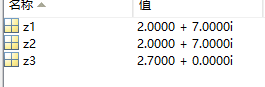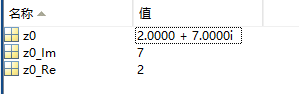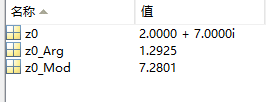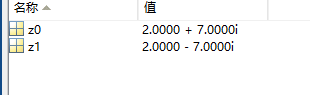• 笔记


z

=

x

+

i

y

z=x+iy

arg

⁡

z

\arg z

x

<

0

x<0

x

=

0

x=0

x

>

0

x>0

y

<

0

y<0

arctan

⁡

y

x

−

π

\arctan\frac{y}{x}-\pi

−

1

2

π

-\frac{1}{2}\pi

arctan

⁡

y

x

\arctan\frac{y}{x}

y

=

0

y=0

π

\pi

0

0

y

>

0

y>0

arctan

⁡

y

x

+

π

\arctan\frac{y}{x}+\pi

1

2

π

\frac{1}{2}\pi

arctan

⁡

y

x

\arctan\frac{y}{x}

2021年3月21日19:32:16
展开全文算法 线性代数
• MATLAB笔记之复数基本公式 QQ:3020889729 小蔡复数的创建复数的实部与虚部的获取复数的辐角与模的获取复数的共轭运算 QQ:30...


MATLAB笔记之复数基本公式
QQ:3020889729                                                                                 小蔡复数的创建复数的实部与虚部的获取复数的辐角与模的获取复数的共轭运算

QQ:3020889729                                                                                 小蔡
复数的创建
% 创建复数
z1 = 2 + 7*1i;% 表达式直接创建
z2 = 2 + 7*i;% 注意了，有时候版本要求，提示需要将*1i代替i来避免错误
z3 = complex(2.7);% 创建实部为2，虚部为7的的复数

效果：复数的实部与虚部的获取
% 复数的实部与虚部的获取
z0 = complex(2,7);% 创建实部为2，虚部为7的的复数
z0_Im = imag(z0);% 用imag获取复数的虚部
z0_Re = real(z0);% 用real获取复数的实部复数的辐角与模的获取
% 复数的辐角与模的获取
z0 = complex(2,7);% 创建实部为2，虚部为7的的复数
z0_Mod = abs(z0);% 用abs获取复数的模——也可以用来求实数的绝对值
z0_Arg = angle(z0);% 用angle获取复数的辐角（主）值——也可以求其它实数的相位角复数的共轭运算
% 复数的共轭运算
clear,clc;
z0 = complex(2,7);% 创建实部为2，虚部为7的的复数
z1 = conj(z0);% 取得指定复数的(复)共轭复数展开全文matlab
• 欧拉公式求长期率的matlab代码火炬 复杂张量和复杂函数的Pytorch扩展。 受启发。 根据论文： 深度复杂网络（） ary进化递归神经网络（） 关于复值卷积神经网络（） 表中的内容： 职能 exp（z） 对数（z） 罪（z） ...
• 欧拉复数公式欧拉复数公式欧拉公式泰勒级数展开近似值 欧拉复数公式 公式： eiπ+1=0e^{i\pi}+1 = 0eiπ+1=0 这个方程真的很奇妙，因为它集合了： eee （欧拉数） iii （单位 虚数) π\piπ （大名鼎鼎的 pi，一个...


欧拉复数公式
欧拉公式泰勒级数展开近似值
欧拉公式，复数域的成人礼总结

公式：

e

i

π

+

1

=

0

e^{i\pi}+1 = 0

这个方程真的很奇妙，因为它集合了：

e

e

（欧拉数）

i

i

（单位 虚数)

π

\pi

（大名鼎鼎的 pi，一个在很多不同领域都出现的数）0 和 1（也是不凡的数！）
欧拉公式
这方程其实源自欧拉公式：

e

i

x

=

cos

⁡

x

+

i

sin

⁡

x

e^{ix} = \cos x + i \sin x

以

x

=

π

x = π

，我们得到：

e

i

π

=

cos

⁡

π

+

i

sin

⁡

π

e

i

π

=

−

1

+

i

×

0

（

因

为

cos

⁡

π

=

−

1

和

sin

⁡

π

=

0

）

e

i

π

=

−

1

e

i

π

+

1

=

0

\begin{aligned} &e^{iπ} = \cos π + i \sin π\\ &e^{iπ} = −1 + i × 0 （因为 \cos π = −1 和 \sin π = 0）\\ &e^{iπ} = −1\\ &e^{iπ} + 1 = 0 \end{aligned}

故此，

e

i

π

+

1

=

0

e^{iπ} + 1 = 0

只不过是更有用的欧拉公式的一个特例。
我们可以把任何点（例如

3

+

4

i

3 + 4i

）变成

r

e

i

x

re^{ix}

的格式（只需找到

x

x

的值和圆形的半径，

r

r

）
例子：

3

+

4

i

3 + 4i

把这复数转换为

r

e

i

x

re^{ix}

的格式，我们要转换笛卡尔坐标为极坐标：

r

=

(

3

2

+

4

2

)

=

(

9

+

16

)

=

25

=

5

r = \sqrt{(3^2 + 4^2)} = \sqrt{(9+16)} = \sqrt{25} = 5

x

=

arctan

⁡

(

4

/

3

)

=

0.927

（

保

留

三

位

小

数

）

x = \arctan( 4 / 3 ) = 0.927 （保留三位小数）

所以

3

+

4

i

3 + 4i

也可以是

5

e

0.927

i

5e^{0.927 i}

。
未完待续…
From: 欧拉复数公式
泰勒级数展开
泰勒级数展开总和符号记法

e

x

=

1

+

x

+

x

2

2

!

+

x

3

3

!

+

⋯

e^{x} = 1+ x + \frac{x^2}{2!} + \frac{x^3}{3!} + \cdots

∑

n

=

0

∞

x

n

n

!

\sum_{n=0}^{\infty} \frac{x^n}{n!}

sin

⁡

x

=

x

−

x

3

3

!

+

x

5

5

!

−

⋯

\sin x = x - \frac{x^3}{3!} + \frac{x^5}{5!} - \cdots

∑

n

=

0

∞

(

−

1

)

n

(

2

n

+

1

)

!

x

2

n

+

1

\sum_{n=0}^{\infty} \frac{(-1)^n}{(2n+1)!}x^{2n+1}

cos

⁡

x

=

1

−

x

2

2

!

+

x

4

4

!

−

⋯

\cos x = 1 - \frac{x^2}{2!} + \frac{x^4}{4!} - \cdots

∑

n

=

0

∞

(

−

1

)

n

(

2

n

)

!

x

2

n

\sum_{n=0}^{\infty} \frac{(-1)^n}{(2n)!}x^{2n}

1

1

−

x

=

1

+

x

+

x

2

+

x

3

+

⋯

f

o

r

∥

x

∥

<

1

\frac{1}{1-x} = 1 + x + x^2 + x^3 + \cdots \quad for\ \|x\|<1

∑

n

=

0

∞

x

n

\sum_{n=0}^{\infty} x^n

近似值
你可以用泰勒级数的头几项来估计函数的近似值。
这里是越来越准确的 cos(x) 近似值。红线是 cos(x)，蓝线是近似值（自己画图来看看）：
From: 泰勒级数展开
欧拉公式，复数域的成人礼
但向量没有乘法（点积、叉积和实数乘法不一样），这就是复数和向量的区别。复数是对实数的扩展，所以要尽量兼容实数，必须要有加减乘除、乘方开方、对数等运算。
可能你还会问，直接替换

x

x

为

i

θ

i\theta

，合理吗：这里是理解欧拉公式的 关键 ，我们要意识到一点，欧拉公式是一种人为的选择，完全可以不这么去定义

e

i

θ

e^{i\theta}

。但是，做了别的选择，会面临一个问题：会不会在现有的庞大复杂的数学体系中产生矛盾？
打个比方吧，在实数中“除以0 ”是不合理的，假如你想让它变得合理，那么分分钟会导出矛盾：欧拉公式并不会引发冲突，并且随着学习的深入，你会发现数学家已经证明了它是一种足够好的选择，这里就不赘述了。
总结
有了欧拉公式后，任何复数都可以表示为：

z

=

a

+

b

i

=

r

e

i

θ

z = a + bi = r e^{i\theta}

其中：

r

=

∣

z

∣

,

θ

=

a

r

g

(

z

)

个人觉得

a

+

b

i

a+bi

只是复数的初始形态，而

r

e

i

θ

re^{i\theta}

才是复数的完成形态，因为它更具有启发性。
比如计算乘法时：

z

1

=

r

1

e

i

θ

1

,

z

2

=

r

2

e

i

θ

2

那么有：

z

1

×

z

2

=

r

1

r

2

e

i

(

θ

1

+

θ

2

)

z_1 \times z_2 = r_1r_2e^{i(\theta_1+\theta_2)}

z

1

÷

z

2

=

r

1

r

2

e

i

(

θ

1

−

θ

2

)

z_1 \div z_2 = \frac{r_1}{r_2}e^{i(\theta_1-\theta_2)}

几何意义更加明显。并且扩展了乘方和对数运算：

a

i

=

e

i

ln

⁡

a

a^i=e^{i\ln a}

ln

⁡

i

⏟

单

位

圆

上

幅

角

为

π

2

的

点

=

ln

⁡

(

e

i

π

2

)

=

i

π

2

\ln \underbrace{i}_{单位圆上幅角为\frac{\pi}{2}的点}=\ln \left(e^{i\frac{\pi}{2}}\right)=i\frac{\pi}{2}

到此为止，基本上所有的初等运算都全了。更多高等的运算比如三角函数、积分、导数，也需要借助欧拉公式在复数上进行推广。
欧拉公式中，如果取

θ

=

π

\theta=\pi

，就得到了欧拉恒等式：

e

i

π

+

1

=

0

e^{i\pi}+1=0

这个公式也被誉为了上帝公式，包含了数学中最基本的

e

、

π

、

i

、

1

、

0

e 、\pi 、i 、1 、0

，仿佛一句诗，道尽了数学的美好。
From: 欧拉公式，复数域的成人礼
展开全文欧拉回路 latex 代码规范
• ## 复数与矢量的计算

千次阅读 2010-12-29 14:06:00
这是我写一个与无线计算相关的小工具时而编写的代码，目的是为这完成矢量或复数计算。同时，能完成矢量表达式：《模/相角》与《实数+i实数》这两种形式的自动转换。 代码使用delphi 7.0 完成。对于接口部分，是...
这是我写一个与无线计算相关的小工具时而编写的代码，目的是为这完成矢量或复数的计算。同时，能完成矢量表达式：《模/相角》与《实数+i实数》这两种形式的自动转换。

代码使用delphi 7.0 完成。对于接口部分，是为了导出到其它环境中使用。方式可能不是最好，但目的我找不到更好的方式，权宜用着。

unit my_complex;
{\$WARN SYMBOL_PLATFORM OFF}
interface
uses
Windows, ActiveX, Classes, ComObj, my_typ_TLB, StdVcl;
type TComplexObj=Class(TObject)
protected
Fre:Double;
Fim:Double;
Fmo:Double;
Fan:Double;
procedure SetRe(val:Double);
procedure SetIm(val:Double);
procedure SetMo(val:Double);
procedure SetAn(val:Double);
public
property re:Double read Fre write SetRe;
property im:Double read Fim write SetIm;
property mo:Double read Fmo write SetMo;
property an:Double read Fan write SetAn;
End;
type
TComplex = class(TTypedComObject, IComplex)
protected
{Declare IComplex methods here}
Function _Sub(com1:TComplexObj;com2:TComplexObj):TComplexObj;
Function _Mux(com1:TComplexObj;com2:TComplexObj):TComplexObj;
Function _Div(com1:TComplexObj;com2:TComplexObj):TComplexObj;
function CreateVector(modulus, angle: Double): OLE_HANDLE; stdcall;
function CreateComplex(real, imaginary: Double): OLE_HANDLE; stdcall;
function DestoryObject(aHandle: OLE_HANDLE): WordBool; stdcall;
function Angle(aHandle: OLE_HANDLE): Double; stdcall;
function Real(aHandle: OLE_HANDLE): Double; stdcall;
function Modulus(aHandle: OLE_HANDLE): Double; stdcall;
function Imaginary(aHandle: OLE_HANDLE): Double; stdcall;
function Mux(multiplicand, multiplier_: OLE_HANDLE): WordBool; stdcall;
function Sub(minuend, subtrahend: OLE_HANDLE): WordBool; stdcall;
function Division(dividend, divisor: OLE_HANDLE): WordBool; stdcall;
end;

implementation
uses ComServ,math;
procedure TComplexObj.SetRe(val:Double);
Begin
Fre:=Val;
Fmo:=sqrt(sqr(Fre)+sqr(Fim));
End;
procedure TComplexObj.SetIm(val:Double);
Begin
FIm:=Val;
If (FRe=0) then Exit;
Fmo:=sqrt(sqr(Fre)+sqr(Fim));
End;
procedure TComplexObj.SetMo(val:Double);
Begin
Fmo:=Val;
End;
procedure TComplexObj.SetAn(val:Double);
Begin
FAn:=Val;
End;
begin
result.re:=com1.re+com2.re;
result.im:=com1.im+com2.im;
end;
function TComplex._Sub(com1, com2: TComplexObj): TComplexObj;
begin
result.re:=com1.re-com2.re;
result.im:=com1.im-com2.im;
end;

function TComplex._Mux(com1, com2: TComplexObj): TComplexObj;
begin
end;
function TComplex._Div(com1,com2:TComplexObj):TComplexObj;
var com:TComplexObj;
Begin
com:=com2;
com.im:=0-com.im;
result:=self._Mux(com1,com);
result.re:=result.re /( sqr(com2.re)+sqr(com2.im));
result.im:=result.im /( sqr(com2.re)+sqr(com2.im));
End;
function TComplex.CreateVector(modulus, angle: Double): OLE_HANDLE;
var ptr:TComplexObj;
begin
ptr:=TComplexObj.Create();
try
ptr.SetMo(modulus);
ptr.SetAn(angle);
result:=OLE_HANDLE(ptr);
except
ptr:=nil;
result:=0;
end;
end;
function TComplex.CreateComplex(real, imaginary: Double): OLE_HANDLE;
var ptr:TComplexObj;
begin
try
ptr:=TComplexObj.Create();
ptr.SetRe(real);
ptr.SetIm(imaginary);
result:=OLE_HANDLE(ptr);
except
ptr:=nil;
result:=0;
end;
end;
function TComplex.DestoryObject(aHandle: OLE_HANDLE): WordBool;
begin
try
TComplexObj(aHandle).Free;
result:=true;
except
result:=false;
end;
end;

function TComplex.Angle(aHandle: OLE_HANDLE): Double;
begin
result:=TComplexObj(aHandle).an;
end;
function TComplex.Real(aHandle: OLE_HANDLE): Double;
begin
result:=TComplexObj(aHandle).re;
end;
function TComplex.Modulus(aHandle: OLE_HANDLE): Double;
begin
result:=TComplexObj(aHandle).mo;
end;
function TComplex.Imaginary(aHandle: OLE_HANDLE): Double;
begin
result:=TComplexObj(aHandle).im;
end;
var aComplexObj:TComplexObj;
begin
try
TComplexObj(augend):=aComplexObj;
result:=true;
except
result:=false;
end;
end;
function TComplex.Mux(multiplicand, multiplier_: OLE_HANDLE): WordBool;
var aComplexObj:TComplexObj;
begin
try
aComplexObj:=self._Mux(TComplexObj(multiplicand),TComplexObj(multiplier_));
TComplexObj(multiplicand):=aComplexObj;
result:=true;
except
result:=false;
end;
end;
function TComplex.Sub(minuend, subtrahend: OLE_HANDLE): WordBool;
var aComplexObj:TComplexObj;
begin
try
aComplexObj:=self._Sub(TComplexObj(minuend),TComplexObj(subtrahend));
TComplexObj(minuend):=aComplexObj;
result:=true;
except
result:=false;
end;
end;
function TComplex.Division(dividend, divisor: OLE_HANDLE): WordBool;
var aComplexObj:TComplexObj;
begin
try
aComplexObj:=self._Div(TComplexObj(dividend),TComplexObj(divisor));
TComplexObj(dividend):=aComplexObj;
result:=true;
except
result:=false;
end;
end;
initialization
TTypedComObjectFactory.Create(ComServer, TComplex, Class_Complex,
ciMultiInstance, tmApartment);
end.

展开全文function delphi class methods div
• 一、计算方式 二、备注 1.DeepGraphLearning/KnowledgeGraphEmbedding 2.The calculation of complex vector线性代数
• 福利彩票中的双色球复式算法，算法关键之处在于键值跟数值和数组合并复式算法
• 利用mathematica计算复数的对数难以找到合适的帮助文档，有意思的是找了基本数学手册居然没有复数对数的普遍运算公式，真是奇怪。不得不自己动手弄出来，本人将复数的各种运算包括指数特别是对数的运算方法和技巧写...
• ## 欧拉公式，复数域的成人礼

千次阅读 多人点赞 2018-12-19 14:17:30
之前在“复数，通往真理的最短路径”中说过，复数域其实就是二维的数域，提供了更高维度的、更抽象的视角。本文来看看，我们是怎么从实数域扩展到复数域的。 大家可能觉得这个扩展并不复杂，也就是 、 两个任意...
• 式中sgn表示符号函数，即sng(x)={1 if x>00 if x=0−1 if x<0sng(x)= \begin{cases} 1 & \text{ if } x>0 \\ 0 & \text{ if } x=0 \\ -1 &...x.
• 主要内容：复数与复变函数、解析函数、复变函数的积分、级数、留数、保形映射、积分变换等。 一、复数基本知识 1.1 复数基本概念 对任意两实数x, y，称z=x+iyz=x+iyz=x+iy或z=x+yiz=x+yiz=x+yi为复数，其中i2=−1i^...复变函数
• 设有两个复数 和 ，则他们的运算公式为：　要求：（1）定义一个结构体类型来描述复数。　（2）复数之间的加法、减法、乘法和除法分别用不用的函数来实现。　（3）必须使用结构体指针的方法把函数的计算结果返回。　...
• 感抗和容抗公式  实验证明，感抗和电感成正比，和频率也成正比。如果感抗用XL表示，电感用L表示，频率用f表示，那么感抗公式为： 。 交流电也可以通过线圈，但是悬泉的电感对交流电有阻碍作用，这个阻碍就叫做...滤波
• Ez是一个关于phi和z的复数矩阵，假设行表示phi，列表示z，phi取值范围为-180~180(2度一个点)，n取值范围为-90~90，z取值范围为-2.5~2.5（0.05一个点），h取值范围为-50~50,那么我根据公式求和运算计算结果和直接...fft matlab
• 选择实数或者复数进行运算，手动输入数据，然后进行加减乘除操作运算，输出结果
• 复数： z = x + jy. 通常在平面坐标中表示， x轴为实轴， y轴为虚轴。 复数加法和减法是实轴和实轴相加，虚轴和虚轴相加。 乘法： 欧拉公式： e^iθ = cos(θ) + j sin(θ) 当 θ = π ， e^iπ = -1 ...
• 复数表达形式输入两个复数，选择要进行的运算。会以复数表达形式显示其运算结果。
• ## Java实现复数运算

万次阅读 多人点赞 2019-07-21 21:44:59
设有两个复数 和 ，则他们的运算公式为： 要求：（1）定义一个结构体类型来描述复数。 　（2）复数之间的加法、减法、乘法和除法分别用不用的函数来实现。 　（3）必须使用结构体指针的方法把函数的计算结果返回。 ...Java
• 今天接触到了量子计算，原理正在摸索，但是从数学角度，复数计算应该会用到。 以前学习复数的时候，纯属应付考试，现在回想只记得一个公式：，是的，就只记得这个！ 今天周末，有时间缕一下，看几个问题： 1、...
• 数学物理方法·基础⑦基本初等复变函数的计算公式/方法 QQ:3020889729 小蔡复幂函数（指数为实数）复指数函数复三角函数反三角函数复反双曲函数复根式函数复对数函数一般复幂函数（指数为复...
• 2、有一个测试函数，计算器会随机出复数的加减乘的运算题给用户做，答对可以等分，做完所有题目，计算器会计算出用户最终的得分，还有平均得分。 3、用户的操作会记录在一个文件中，下次使用计算器会有相应的记录，...c++
• ## python~计算公式的值

千次阅读 2020-04-11 14:19:12
代码： import math i = int(input()) m = 15/180*math.pi res = math.sin(m)+\ (math.exp(i) - 5 * i)/math.sqrt(i**2+1) - math.log(3*...cmath是针对复数计算的，结构为复数，复数是不可调用round（）函数将其规...python
•python 编程语言
• 欧拉公式：e^ix=cosx+isinx ∵将e^ix按泰勒展开得e^x=1+x/1!+x^2/2!+x^3/3!+x^4/4!+……  将cos x按泰勒展开得cos x=1-x^2/2!+x^4/4!-x^6/6!……  将sin x按泰勒展开得sin x=x-x^3/3!+x^5/5!-x^7/7!……  转换到...
• 证明欧拉公式 如果这么看自变量：θ=ωt\theta= \omega t θ=ωt那么就可以发现欧拉公式的几何意义。 复数的表示形式 通过下面对比可以发现，用复指数表示复数在几何上更直观点。 复数的运算 1.加法运算 设z1=a+...
• ## 复数的运算法则

千次阅读 2021-01-21 14:52:27
复数的加法法则：设z1=a+bi，z2=c+di是任意两个复数。两者和的实部是原来两个复数实部的和，它的虚部是原来两个虚部的和。两个复数的和依然是复数。即 2.乘法法则 复数的乘法法则：把两个复数相乘，类似两个多项式...抽象代数
• 复数计算题目（ P1103） 编程实现两个复数的运算。设有两个复数 和 ，则他们的运算公式为： 要求：（1）定义一个结构体类型来描述复数。 　（2）复数之间的加法、减法、乘法和除法分别用不用的函数来实现。 　（3）...算法 指针 经验分享
• 计算双色球复式（单式也按复式计算，胆拖可先拆成复式来计算）的算法就是一个简单的排列组合算法。 例如：  选了m个红球，中了n个红球，选了k个篮球，中了j个篮球（m、n、k、j数值限制请参考上面玩法介绍）  ...java Java JAVA
• 无线传输距离计算 Pr(dBm) = Pt(dBm) - Ct(dB) + Gt(dB) - FL(dB) + Gr(dB) - Cr(dB) Pr：接受端灵敏度 Pt: 发送端功率 Cr: 接收端接头和电缆损耗 Ct: 发送端接头和电缆损耗 Gr: 接受端天线增益 Gt: 发送...
• 作者：桂。 时间：2018-01-2719:52:57 链接：... 前言 ...本文摘自：《复数信号模值近似计算及硬件实现》 正文 硬件具体实现流程不再赘述。 ......